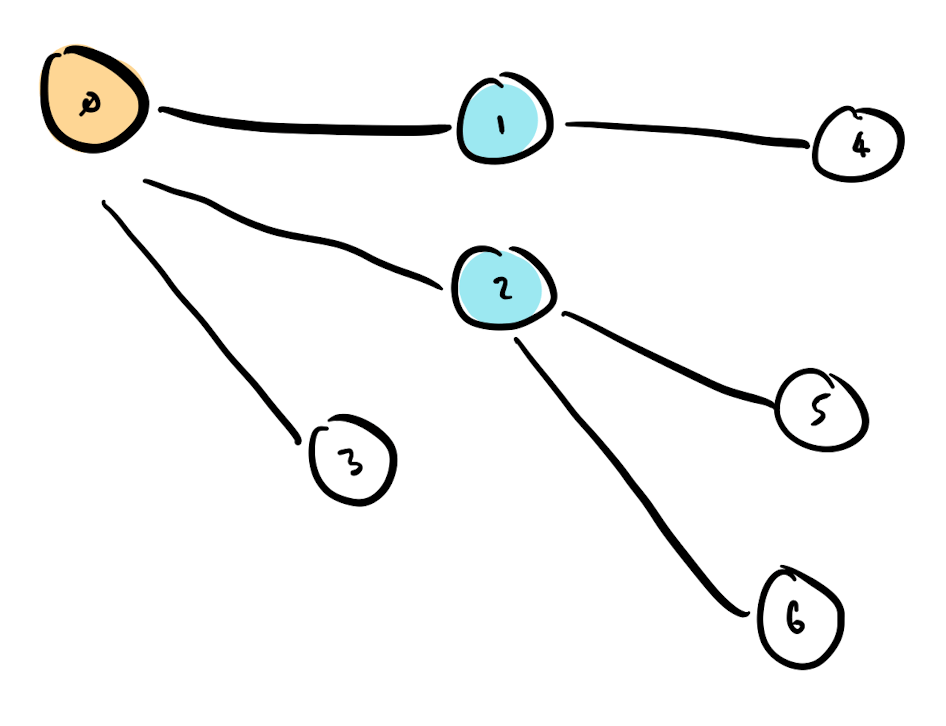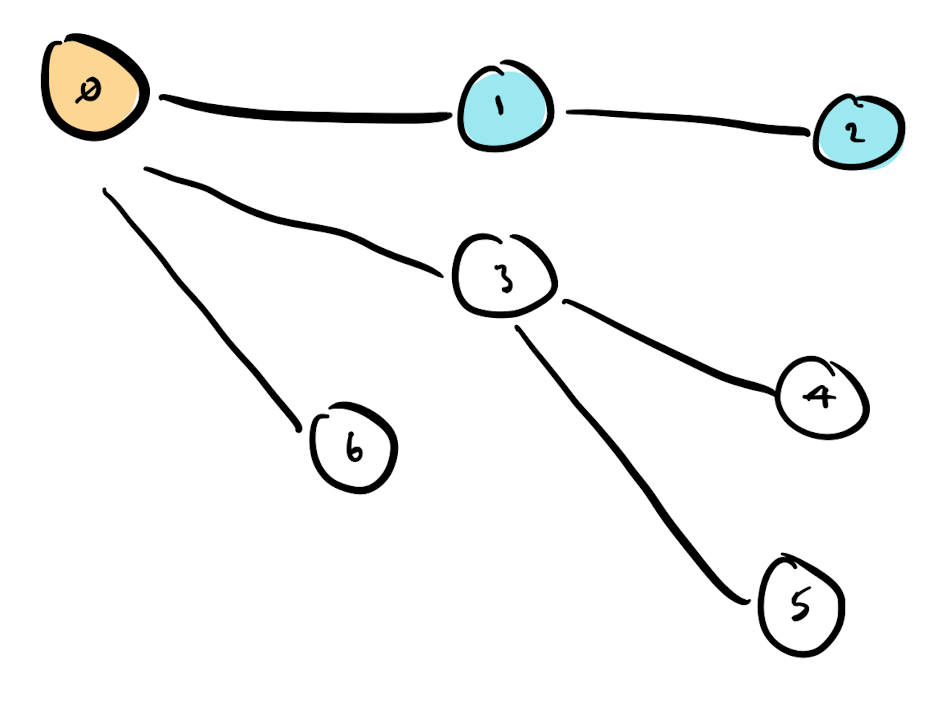# Depth First Search vs Breadth First Search - A Recap

Depth First Search (DFS) and Breadth First Search (BFS) are two searching algorithms that traverse tree or graph data structures.

They are very similar in implementation and only differ in the choice of which node to visit next.

## BFS

Visit a node, find connected nodes, and then visit them all in turn.

BFS is easy to describe as the order in which nodes are visited can be modelled as a queue.

We start at node zero, and find three connected nodes `[1, 2, 3]`. We visit each in turn, storing their respective connections in the queue.

The image below has numbered labels to show the order in which nodes are visited. Node zero, plus the first two visited are coloured.## DFS

Visit a node, find connected nodes, and then visit them first. Newly discovered nodes are visited as a priority.

The image below again shows the order in which the nodes are visited. The order has changed. The second node visited is connected to the first node.## Queue vs Stack

As mentioned, BFS can be implemented with a Queue. Nodes are visited in the order they are discovered. This is First-In-Last-Out (FIFO) behaviour.

DFS is Last-In-First-Out (LIFO) behaviour. This can be implemented with a Stack.

## Implementation (with Python)

Given the Python tree structure below, we can implement simple algorithms for BFS and DFS that traverse the tree, and return the label of the nodes visited.

``````tree = Node("Origin", connected = [
Node("A", connected=[
Node("D")
]),
Node("B", connected=[
Node("E"),
Node("F")
]),
Node("C")
])
``````

The code below uses a standard Python list as the queue, and implements BFS.

We pop from the left, and append to the right.

``````def bfs(tree):
visited = []
queue = [tree]

while queue:
# get a node from the queue.
current = queue.pop(0)
# add node to the visited list.
visited.append(current.val)
# add connections to the queue.
for c in current.connected:
queue.append(c)

return visited
``````

In practice using `collections.deque` is more efficient than this code, as `list.pop(0)` has a time complexity of `O(n)` due to every element in the list being shifted left.

We can alter this code to implement a DFS algorithm. Instead of `.pop(0)` we can use `pop()`. That is the only change we need, and the time complexity of `pop()` is `O(1)` now.

Pop from the right, and append to the right; a classic stack datastructure.

``````def dfs(tree):
visited = []
stack = [tree]

while stack:
current = stack.pop()
visited.append(current.val)
for c in current.connected:
stack.append(c)

return visited
``````

The interesting thing about this implementation is that 'Node C' is visited first. As we push the connected nodes on the stack, we first have a Stack of `[A, B, C]`.

To visit 'Node A' first, we need to reverse the `current.connected` list before pushing to the stack... or implement a simple recursive solution instead:

``````def dfs_recursive(tree, visited = []):
visited.append(tree.val)
for c in tree.connected:
dfs_recursive(c, visited)
return visited
``````